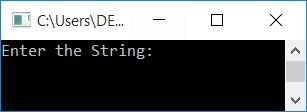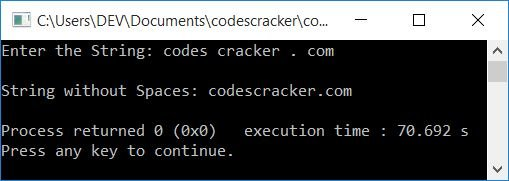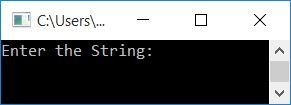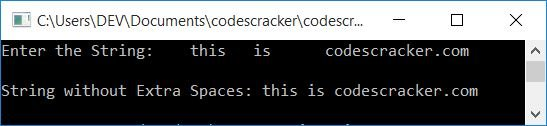# Remove All and Extra Spaces from a String in C++

In this article, you will learn and get code to remove spaces from strings using a C++ program. Here is the list of programs that delete or remove spaces from strings entered by the user at run-time:

In both programs, a string must be entered by the user at run-time.

## Remove all spaces from the string in C++

To remove or delete all spaces from a string or a sentence, you have to ask the user to enter the string. Then, if a space is found, shift all the characters one index back from where it was found, as shown in the program below:

The question is, "Write a program in C++ to remove all spaces from strings." Here is its answer:

```#include<iostream>
#include<stdio.h>
using namespace std;
int main()
{
char str;
int i=0, j, chk;
cout<<"Enter the String: ";
gets(str);
while(str[i]!='\0')
{
chk=0;
if(str[i]==' ')
{
for(j=i; str[j-1]!='\0'; j++)
str[j] = str[j+1];
chk = 1;
}
if(chk==0)
i++;
}
cout<<"\nString without Spaces: "<<str;
cout<<endl;
return 0;
}```

This program was built and runs under the Code::Blocks IDE. Here is its sample run:Now enter any string, such as codes cracker . com, and press the ENTER key to remove all spaces and print the same string without any spaces, as shown in the screenshot below:The dry run of the above program with user input codes cracker . com goes like:

• When the user enters the string, then it gets stored in the str variable in a way that:
• str=c
• str=o
• str=d
• str=e
• str=s
• str=' '
• str=c
• str=r
• and so on up until
• str=m
• And at the last index, that is, the 19th index, a null-terminated character (\0) automatically gets assigned. So str='\0'
• Because the initial value of i is 0. Therefore, the condition of the while loop str[i]!='\0' or str!='\0' or c!='\0' evaluates to be true, therefore program flow goes inside the loop.
• Inside the loop, 0 gets initialized to chk. So chk=0
• Now that the condition of if, str==' ' or c==' ' evaluates to false, therefore program flow evaluates the condition of second if.
• That is, the condition chk==0 or 0==0 evaluates to be true, therefore program flow goes inside the if's body and increments the value of i. So i=1
• Now program flow evaluates the condition of the while loop again. That is, the condition str[i]!='\0' or str!='\0' or o!='\0' evaluates to be true again, therefore program flow goes inside the loop again.
• This process is repeated until the condition of the while loop evaluates to false.
• If any space occurs, then from the index where a space is found, we've shifted all the characters one index back. For example, if a space is found at index no.5, then:
• character at the 6th index gets moved to the 5th index
• character at the 7th index gets moved to the 6th index
• character at the 8th index gets moved to the 7th index
• and so on.
• After shifting all the characters, we've initialized 1 to chk, to see if it maintains its previous value of 0. If it holds its previous value, then increments the value of i; otherwise, don't increment the value of i, and start from the same (previous) index, or the last evaluation's index.
• Because, through the example of shifting characters above, we've shifted the character at the 6th index to the 5th index, that is now a new character. That is why, we've used the chk variable to check and increment i's value.
• In this way, all the spaces get removed from the string, str. So, after completing the task, simply print its value.

## In C++, remove extra spaces from a string

This program removes only extra spaces from the given string. For example, if there are more than one spaces available between two words, then all extra spaces will be removed. Let's have a look at the program:

```#include<iostream>
#include<stdio.h>
#include<string.h>
using namespace std;
int main()
{
char str;
int i, j, len;
cout<<"Enter the String: ";
gets(str);
len = strlen(str);
for(i=0; i<len; i++)
{
if(str==' ')
{
for(i=0; i<(len-1); i++)
str[i] = str[i+1];
str[i] = '\0';
len--;
i=-1;
continue;
}
if(str[i]==' ' && str[i+1]==' ')
{
for(j=i; j<(len-1); j++)
{
str[j] = str[j+1];
}
str[j] = '\0';
len--;
i--;
}
}
cout<<"\nString without Extra Spaces: "<<str;
cout<<endl;
return 0;
}```

Here is the initial output produced by this program:Now supply any string that contains more than one space between two words, and press `ENTER` to remove extra spaces from the given string and print the new string as shown in the snapshot given below:#### The same program in different languages

C++ Quiz

« Previous Program Next Program »